# CBSE Solutions for Class 10 Maths

#### Select CBSE Solutions for class 10 Subject & Chapters Wise :

If the sum of zeroes of the quadratic polynomial 3x2 – kx + 6 is 3, then find the value of k. (2012)

Answer :

Here p = 3, q = -k, r = 6
Sum of the zeroes, (α + β) = -q/p= 3 …..(given)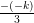= 3
k = 9

If α and β are the zeroes of the polynomial ay2 + by + c, find the value of α2 + β2.

Answer :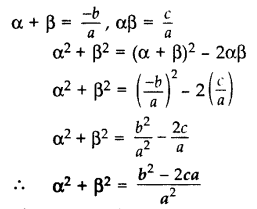If the sum of the zeroes of the polynomial p(x) = (k– 14) x2 – 2x – 12 is 1, then find the value of k. (2017 D)

Answer :

p(x) = (k2 – 14) x2 – 2x – 12
Here a = k2 – 14, b = -2, c = -12
Sum of the zeroes, (α + β) = 1 …[Given in question]
⇒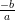= 1
⇒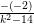= 1
⇒ k2 – 14 = 2
⇒ k2 = 16
⇒ k = ±4

If α and β are the zeroes of a polynomial such that α + β = -6 and αβ = 5, then find the polynomial. (2016 D)

Answer :

Quadratic polynomial is x2 – (Sum)x + (Product) = 0
⇒ x2 – (-6)x + 5 = 0
⇒ x2 + 6x + 5 = 0

Question 5.
A quadratic polynomial, whose zeroes are -4 and -5, is …. (2016 D)

Answer :

x2 + 9x + 20 is the required polynomial.

Find the condition that zeroes of polynomial p(x) = ax2 + bx + c are reciprocal of each other. (2017 OD)

Answer :

Let α and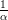be the zeroes of P(x).
P(a) = ax2 + bx + c …(given in the question)
Product of zeroes =⇒ α ×=⇒ 1 =⇒ a = c
Coefficient of x2 = Constant term

Form a quadratic polynomial whose zeroes are 3 + √2 and 3 – √2. (2012)

Answer :

Sum of zeroes = (3 + √2) + (3 – √2) = 6
Product of zeroes = (3 + √2) x (3 – √2) = (3)2 – (√2)2 = 9 – 2 = 7
Quadratic polynomial = x2 – 6x + 7

Find a quadratic polynomial, the stun and product of whose zeroes are √3 and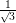respectively. (2014)

Answer :

Sum of zeroes = √3
Product of zeroes =Quadratic polynomial =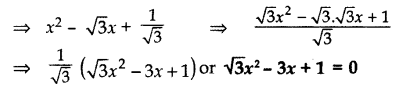Find a quadratic polynomial, the sum and product of whose zeroes are 0 and -√2 respectively. (2015)

Answer :

Quadratic polynomial is
x2 – (Sum of zeroes) x + (Product of zeroes)
= x2 – (0)x + (-√2)
= x2 – √2

Divide 4x3 + 2x2 + 5x – 6 by 2x2 + 1 + 3x and verify the division algorithm. (2013)

Answer :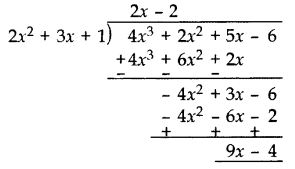Divisor [D]= (2x2 + 3x + 1)

Quotient [Q] = (2x – 2)
Remainder [R] = (9x – 4)
Verification:
[D] × [Q] + [R]
= (2x2 + 3x + 1) × (2x – 2) + 9x – 4
= 4x3 – 4x2 + 6x2 – 6x + 2x – 2 + 9x – 4
= 4x3 + 2x2 + 5x – 6
= Dividend

Given that x – √5 is a factor of the polynomial x3 – 3√5 x2 – 5x + 15√5, find all the zeroes of the polynomial. (2012, 2016)

Answer :

Let,the polynomial  P(x) = x3 – 3√5 x2 – 5x + 15√5
x – √5 is a factor of the given polynomial.
Put x = -√5,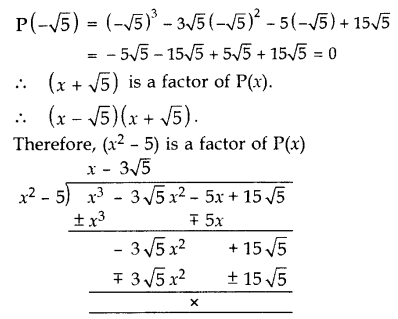Another zero:
x – 3√5 = 0 x = 3√5
All the zeroes of P(x) are -√5, √5 and 3√5.

If a polynomial x4 + 5x3 + 4x2 – 10x – 12 has two zeroes as -2 and -3, then find the other zeroes. (2014)

Answer :

Given two zeroes are -2 and -3.
(x + 2)(x + 3) = x2 + 3x + 2x + 6 = x2 + 5x + 6
Dividing the given equation with x2 + 5x + 6, we get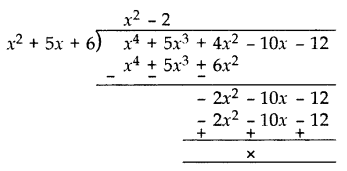x4 + 5x+ 4x2 – 10x – 12
= (x2 + 5x + 6)(x2 – 2)
= (x + 2)(x + 3)(x – √2 )(x + √2 )
Other zeroes are:
x – √2 = 0 or x + √2 = 0
x = √2 or x = -√2

Find all the zeroes of the polynomial 8x4 + 8x3 – 18x2 – 20x – 5, if it is given that two of its zeroes are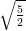and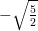. (2014, 2016 D)

Answer :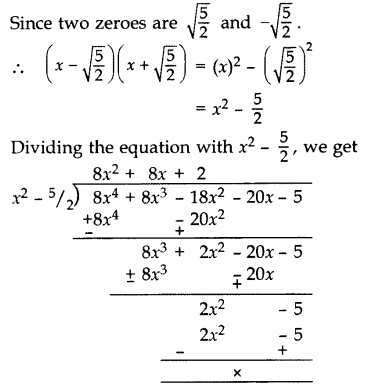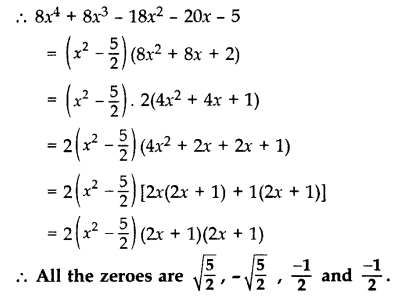If p(x) = x3 – 2x2 + mx + 5 is divided by (x – 2), the remainder is 11. Find m. Hence find all the zeroes of x3 + mx2 + 3x + 1.

Answer :

p(x) = x3 – 2x2 + mx + 5,
When x – 2,
p(2) = (2)3 – 2(2)2 + m(2) + 5
11 = 8 – 8 + 2m + 5
11 – 5 = 2m
6 = 2m
m = 3
Let q(x) = x3 + mx2 + 3x + 1
= x3 + 3x2 + 3x + 1
= x3 + 1 + 3x2 + 3x
= (x)3 + (1)3 + 3x(x + 1)
= (x + 1)3
= (x + 1) (x + 1) (x + 1) …[ a3 + b3 + 3ab (a + b) = (a + b)3]
All zeroes are:
x + 1 = 0 x = -1
x + 1 = 0 x = -1
x + 1 = 0 x = -1
Hence zeroes are -1, -1 and -1.

Degree of polynomial

 1 x5 +x -25 A 0 2 (x+1)(x3 + x2 + 31) B 2 3 9 C 5 4 x2+1 D 4

Answer :

1-C, 2-D, 3-A, 4-B

Equations and Root

 1 X2+ 10x + 25 A -5 & 5 2 X2-5x-50 B 5 & 5 3 X2 -25 C -2 & 20 4 X2+18x-40 D -10 & 5

Answer :

1-B, 2-D, 3-A, 4-C

Equations and Root

 1 X2+ 31x + 30 A -20 & -8 2 X2-28x+160 B -7 & 7 3 X2 -49 C 2 & 3 4 X2+5x+ 6 D 1 & 30

Answer :

1-D, 2-A, 3-B, 4-C

### Take a Test

Choose your Test :

### Chapter 1 : Real Numbers

Polynomials are introduced in Class 9 where we discussed polynomials in one variable and their degrees in the previous class and this is discussed more in detail in Class 10. We study the division algorithm for polynomials of integers and also whether the zeroes of quadratic polynomials are related to their coefficients.

### Browse & Download CBSE Books For Class 10 - All Subjects

The GSEB Books for class 10 are designed as per the syllabus followed Gujarat Secondary and Higher Secondary Education Board provides key detailed, and a through solutions to all the questions relating to the GSEB textbooks.

The purpose is to provide help to the students with their homework, preparing for the examinations and personal learning. These books are very helpful for the preparation of examination.

For more details about the GSEB books for Class 10, you can access the PDF which is as in the above given links for the same.